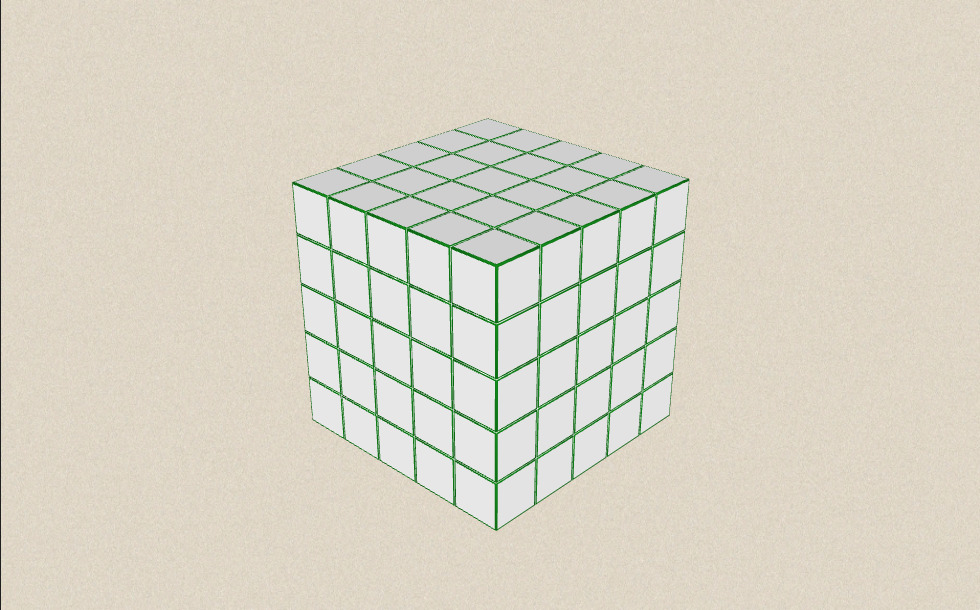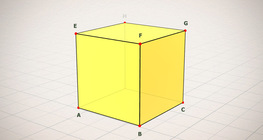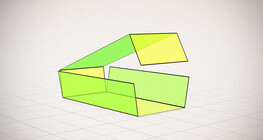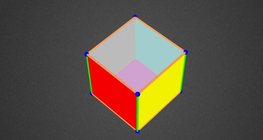Số lượng: 0

Tổng cộng: 0,00

0

# Volume and surface area (exercise)

### Volume and surface area (exercise)

An exercise about the volume and surface area of solids generated from a ´base cube´.

Toán học

Từ khoá

khối lập phương, âm lượng, bề mặt, bài tập

Các mục liên quan

### Các cảnh### Các mục liên quan#### Cube

This animation demonstrates the components (vertices, edges, diagonals and faces) of the cube, one of the Platonic solids.#### Cube puzzle

Building cubes shown in several views from the available unit cubes aids spatial vision and other skills.#### Building shapes (one colour)

Build 3D shapes from unit cubes with the help of several views.#### Cube (exercises)

Edges, diagonals and faces of a cube can be identified by its vertices.#### Cube sections (exercise)

Examining solids formed by the intersection of a cube and a plane.#### Cuboid

A cuboid is a polyhedron with six rectangular faces.#### Net of a cube (exercises)

Not all nets consisting of 6 congruent squares are foldable into cubes.#### Nets of a cuboid (exercises)

This animation demonstrates the different nets of a cuboid and includes a game.#### Notable products

A spectacular demonstration of algebraic expressions.#### Perimeter, area, surface area and volume

This animation presents the formulas to calculate the perimeter and area of shapes as well as the surface area and volume of solids.#### Ratio of volumes of similar solids

This 3D scene explains the correlation between the ratio of similarity and the ratio of volume of geometric solids.#### Volume of spheres (Cavalieri´s principle)

Calculating the volume of a sphere is possible using an appropriate cylinder and cone.#### Volume of spheres (demonstration)

The sum of the volume of the ´tetrahedrons´ gives an approximation of the volume of the sphere.#### Colouring a cube

Colouring the vertices, edges and faces of a given cube according to the criteria specified in the exercise.#### Cube of cubes

An exercise about the regular hexahedron built from unit cubes to help deepen your knowledge of cubes.#### Grouping of cuboids

This animation demonstrates various types of cuboids through everyday objects.#### Non-orientable surfaces

The Möbius strip and the Klein bottle are special two-dimensional surfaces with only one side.